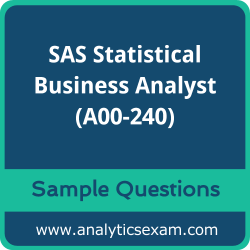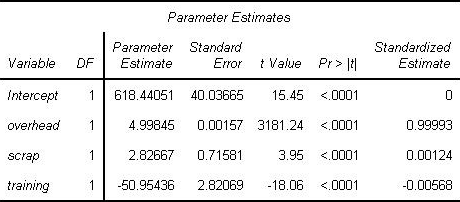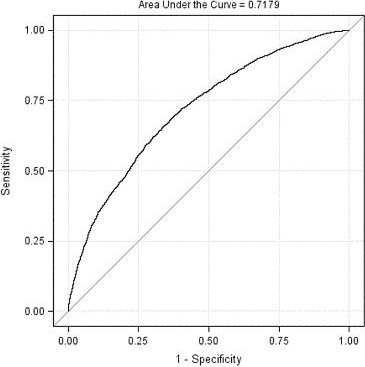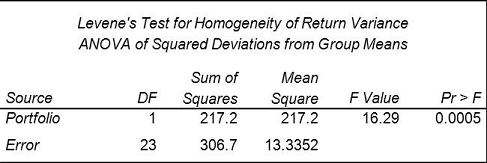# SAS Statistical Business Analyst (A00-240) Certification Exam Sample QuestionsHere are the sample questions which will help you be familiar with SAS Certified Statistical Business Analyst Using SAS 9 - Regression and Modeling (A00-240) exam style and structure. We encourage you to try our Demo SAS Statistical Business Analyst Certification Practice Exam to measure your understanding of exam structure in an environment which simulates the SAS Certified Statistical Business Analyst - Regression and Modeling Certification test environment.

To make your preparation more easy for SAS Certified Statistical Business Analyst - Regression and Modeling (A00-240) exam, we strongly recommend you to use our Premium SAS Statistical Business Analyst Certification Practice Exam. According to our survey with certified candidates, you can easily score 85% in your actual SAS Certification exam if you can score 100% in our premium SAS Statistical Business Analyst Certification practice exams.

## SAS A00-240 Sample Questions:

01. An analyst is screening for irrelevant variables by estimating strength of association between each input and the target variable. The analyst is using Spearman correlation and Hoeffding's D statistics in the CORR procedure.
What would likely cause some inputs to have a large Hoeffding and a near zero Spearman statistic?
a) nonmonotonic association between the variables
b) linear association between the variables
c) monotonic association between the variables
d) no association between the variables

02. Refer to the REG procedure output:What is the most important predictor of the response variable?
a) intercept
c) scrap
d) raining

03. When selecting variables or effects using SELECTION=BACKWARD in the LOGISTIC procedure, the business analyst's model selection terminated at Step 3.
What happened between Step 1 and Step 2?
a) DF increased.
b) AIC increased.
c) Pr > Chisq increased.
d) - 2 Log L increased.

04. A linear model has the following characteristics:
- a dependent variable (y)
- one continuous predictor variables (x1) including a quadratic term (x12)
- one categorical predictor variable (c1 with 3 levels)
- one interaction term (c1 by x1)
Which SAS program fits this model?
a) proc glm data=SASUSER.MLR;
class c1;
model y = c1 x1 x1sq c1byx1 /solution;
run;
b) proc reg data=SASUSER.MLR;
model y = c1 x1 x1sq c1byx1 /solution;
run;
c) proc glm data=SASUSER.MLR;
class c1;
model y = c1 x1 x1*x1 c1*x1 /solution;
run;
d) proc reg data=SASUSER.MLR;
model y = c1 x1 x1*x1 c1*x1;
run;

05. Which statement is an assumption of logistic regression?
a) The sample size is greater than 100.
b) The logit is a linear function of the predictors.
c) The predictor variables are not correlated.
d) The errors are normally distributed.

06. An analyst builds a logistic regression model which is 75% accurate at predicting the event of interest on the training data set. The analyst presents this accuracy rate to upper management as a measure of model assessment.
What is the problem with presenting this measure of accuracy for model assessment?
a) This accuracy rate is redundant with the misclassification rate.
b) It is pessimistically biased since it is calculated from the data set used to train the model.
c) This accuracy rate is redundant with the average squared error.
d) It is optimistically biased since it is calculated from the data used to train the model.

07. Refer to the exhibit:For the ROC curve shown, what is the meaning of the area under the curve?
a) percent concordant plus percent tied
b) percent concordant plus (.5 * percent tied)
c) percent concordant plus (.5 * percent discordant)
d) percent discordant plus percent tied

08. An analyst has determined that there exists a significant effect due to region. The analyst needs to make pairwise comparisons of all eight regions and wants to control the experimentwise error rate.
Which GLM procedure statement would provide the correct output?
a) lsmeans Region / pdiff=all adjust=dunnett;
b) lsmeans Region / pdiff=all adjust=tukey;
c) lsmeans Region / pdiff=all adjust=lsd;
d) lsmeans Region / pdiff=all adjust=none;

09. The LOGISTIC procedure will be used to perform a regression analysis on a data set with a total of 10,000 records. A single input variable contains 30% missing records.
How many total records will be used by PROC LOGISTIC for the regression analysis?
Note:- You can use calculator for this question
a) 7000
b) 9000
c) 7009
d) 9007

10. A financial analyst wants to know whether assets in portfolio A are more risky (have higher variance) than those in portfolio B.
The analyst computes the annual returns (or percent changes) for assets within each of the two groups and obtains the following output from the GLM procedure:Which conclusion is supported by the output?
a) Assets in portfolio A are significantly more risky than assets in portfolio B.
b) Assets in portfolio B are significantly more risky than assets in portfolio A.
c) The portfolios differ significantly with respect to risk.
d) The portfolios do not differ significantly with respect to risk.